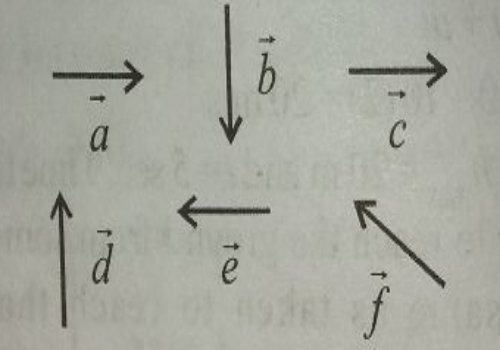# Q&A - Ask Doubts and Get Answers

Sort by :
Clear All
Q
Medical
131 Views   |

Six vectors,  have the magnitudes and directions indicated in the figure. Which of the following statements are true?• Option 1)

• Option 2)

• Option 3)

• Option 4)

As learnt in Commutative law of vector addition - Sum of vector remains the same in whatever order they may be added. Commutative law - wherein Fig. Shows Commutative law of vector addition.   Option 1) This option is incorrect Option 2) This option is incorrect Option 3) This option is correct Option 4) This option is incorrect
Medical
118 Views   |

A particle has initial velocity  and has acceleration . It's speed after 10 s is:

• Option 1)

7 units

• Option 2)

• Option 3)

8.5 units

• Option 4)

10 units

As learnt in

1st equation or velocity time equation -

V = Final velocity

u = Initial velocity

A = acceleration

T = time

-

Option 1)

7 units

This option is incorrect

Option 2)

This option is correct

Option 3)

8.5 units

This option is incorrect

Option 4)

10 units

This option is incorrect

View More
Medical
353 Views   |

A man of 50 kg mass is standing in a gravity free space at a height of 10m above the floor. He throws a stone of 0.5kg mass downwards with a speed of 2m/s. When the stone reaches the floor, the distance of the man above the floor will be:

• Option 1)

9.9m

• Option 2)

10.1m

• Option 3)

10m

• Option 4)

20m

As learnt in Tips of distance - Distance is a scalar quantity. It is always positive. S.I. Unit meter (m). Dimension Distance is depend on path followed by body . - wherein Displacement along circle is zero(0). Semi circle         momentum remains conserved where u - initial velocity of man      (negative sign shows that man moves upward) Time taken by the stone   Distance moved by the...
Medical
254 Views   |

A ball is dropped from a high rise platform at t=0 starting from rest. After 6 seconds another ball is thrown downwards from the same platform with a speed v. The two balls meet at t=18s. What is the value of v? (take g=10m/s2)

• Option 1)

75m/s

• Option 2)

55m/s

• Option 3)

40m/s

• Option 4)

60m/s

As we learnt in 2nd equation or Position- time equation - Displacement Initial velocity acceleration time   -    Distance moved in 18 sec by first half,  Distance moved in 12 sec by second half,  1620 = 12v + 5 x 144 Option 1) 75m/s Correct Option 2) 55m/s Incorrect Option 3) 40m/s Incorrect Option 4) 60m/s Incorrect
Exams
Articles
Questions